## 算法君带你学算法（1）：最长回文字符串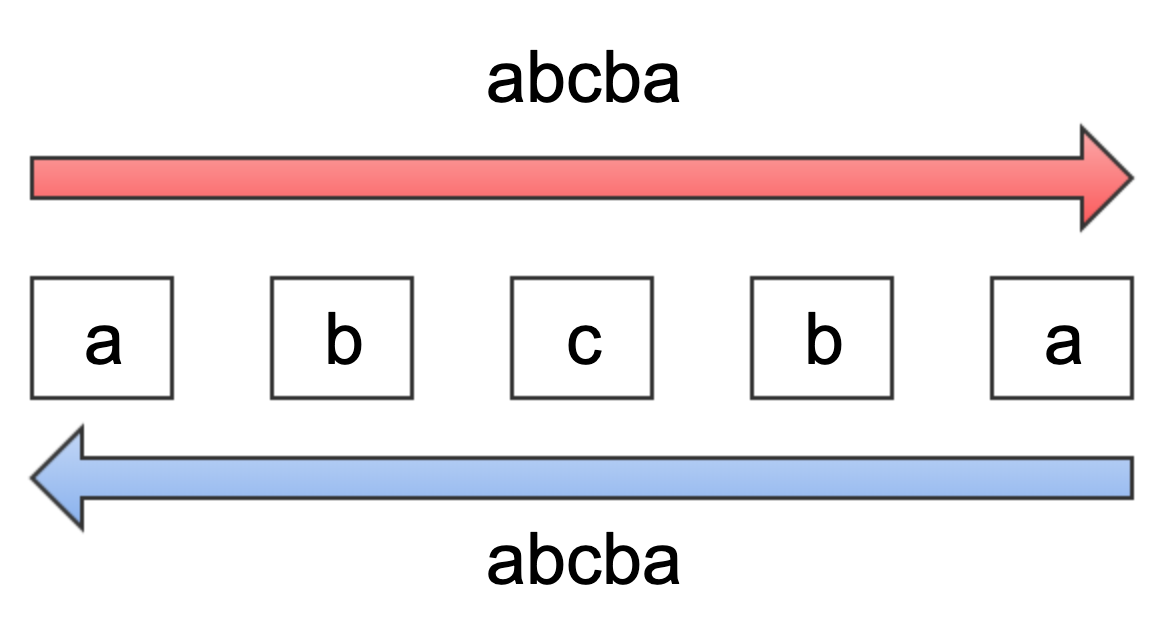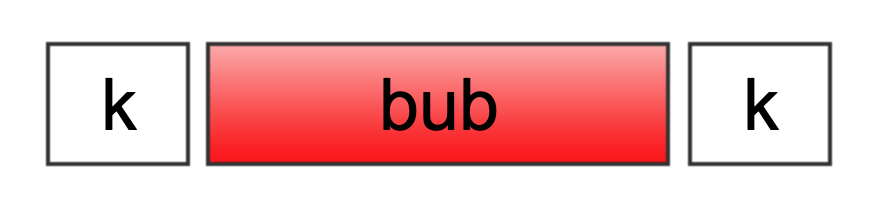1. 判断首尾两个字符是否相同
2. 判断夹在首尾字符中间的子字符串是否为回文字符串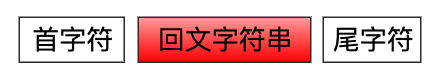history_record[(1,3)]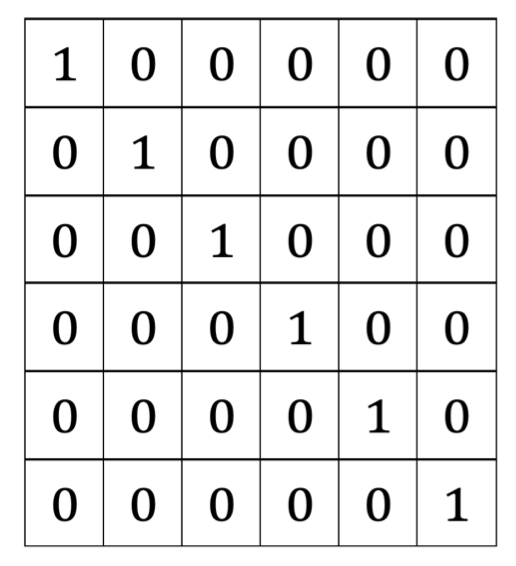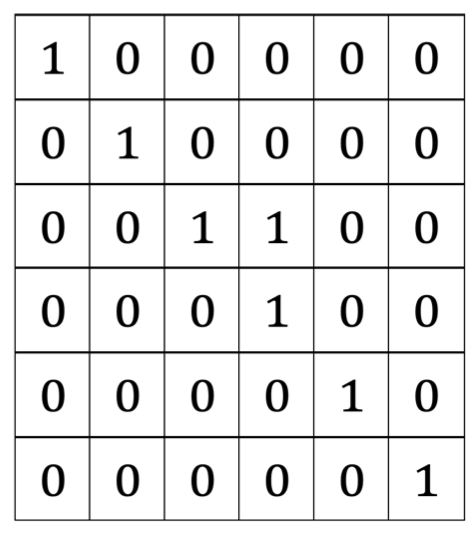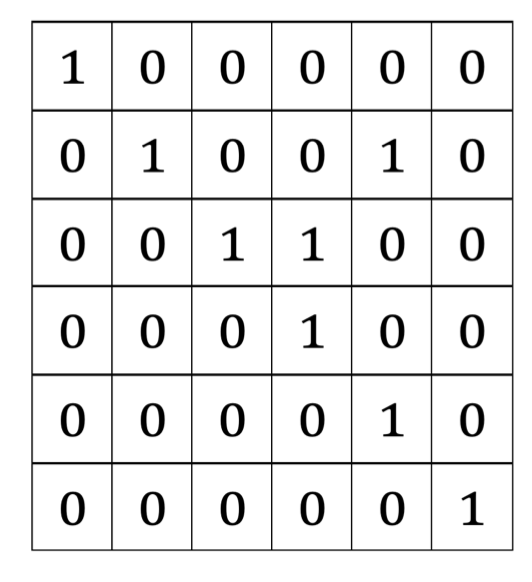#动态规划法求最长回文字符串的完整代码
class MaxPalindromeString:
def __init__(self):
self.start_index = None
self.array_len = None

def get_longest_palindrome(self,s):
if s == None:
return
size = len(s)

if size < 1:
return
self.start_index = 0
self.array_len = 1
# 用于保存历史记录（size * size)
history_record = [( * size) for i in range(size)]

# 初始化长度为1的回文字符串信息
i= 0
while i< size:
history_record[i][i] = 1
i += 1

# 初始化长度为2的回文字符串信息
i = 0
while i < size - 1:
if s[i] == s[i+1]:
history_record[i][i+1] = 1
self.start_index = i
self.array_len = 2
i += 1

# 查找从长度为3开始的回文字符串
p_len = 3
while p_len <= size:
i = 0
while i < size-p_len + 1:
j = i + p_len-1
if s[i] == s[j] and history_record[i+1][j-1] == 1:
history_record[i][j] = 1
self.start_index = i
self.array_len = p_len
i += 1
p_len += 1
s = 'abcdefgfedxyz'
s1 = 'akbubk'
p = MaxPalindromeString()
p.get_longest_palindrome(s1)

if p.start_index != -1 and p.array_len != -1:
print('最长回文字符串：',s1[p.start_index:p.start_index + p.array_len])
else:
print('查找失败')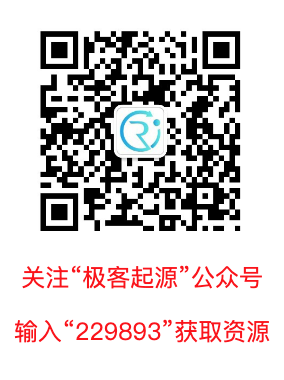posted on 2019-12-24 15:00  银河使者  阅读(726)  评论(0编辑  收藏  举报